# 一块RTX 3090加速训练YOLOv5s，时间减少11个小时，速度提升20%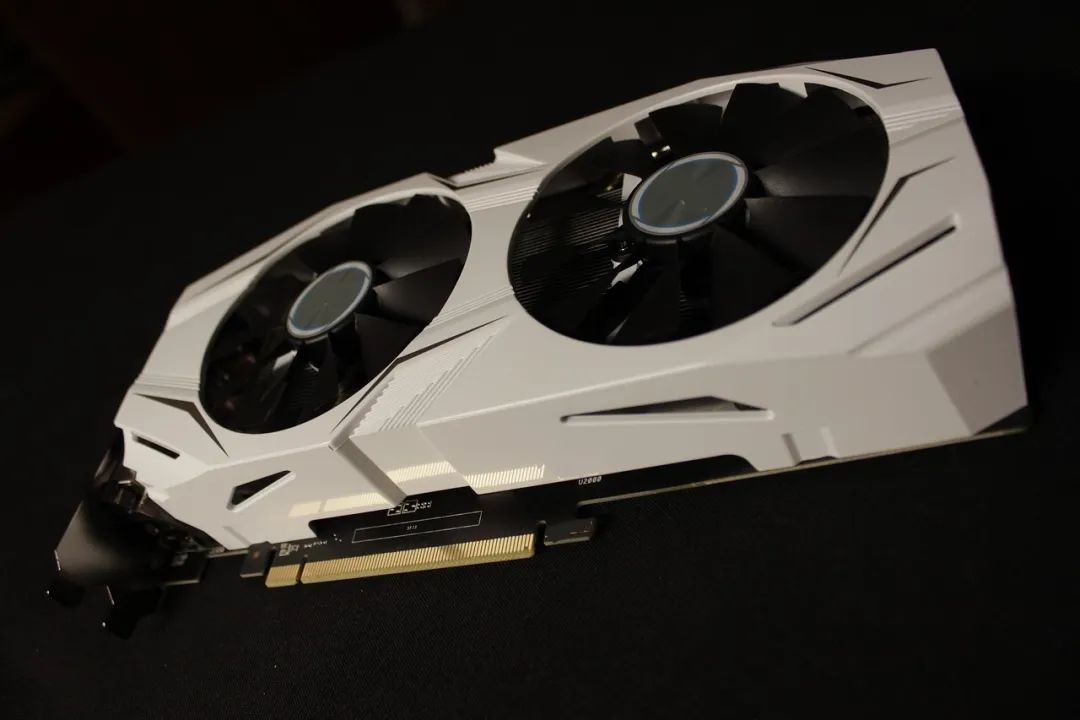#### 不过，可能你也在思考一个问题：虽然OneFlow的兼容性做得很好，可以很方便地移植YOLOv5并使用OneFlow后端来进行训练，但为什么要用OneFlow？能缩短模型开发周期吗？解决了任何痛点吗？本篇文章将尝试回答这几个问题。

One-YOLOv5 链接：
https://github.com/Oneflow-Inc/one-yolov5## 结果展示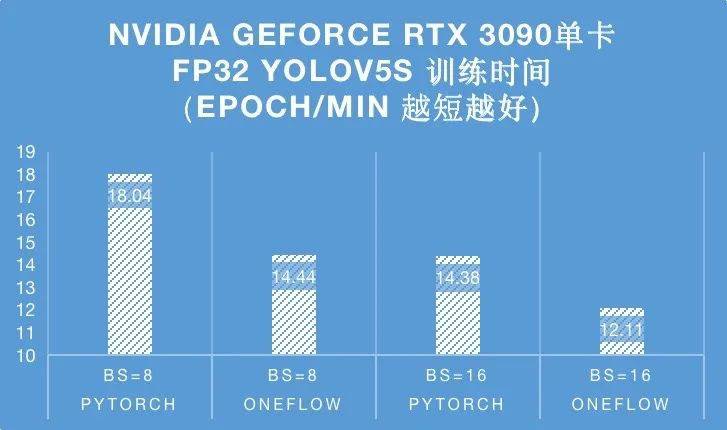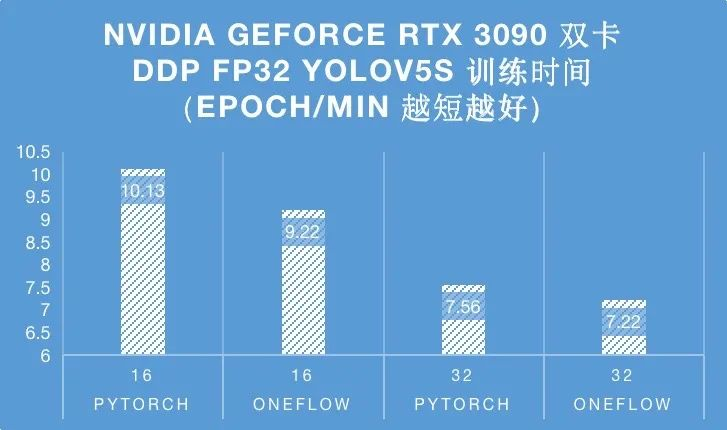## 优化手段

### 2.1 对UpsampleNearest2D的特化改进

````import oneflow as flow`
`x = flow.randn(16, 32, 80, 80, device="cuda", dtype=flow.float32).requires_grad_()`
`m = flow.nn.Upsample(scale_factor=2.0, mode="nearest")`
`y = m(x)``print(y.device)``y.sum().backward()````

https://github.com/Oneflow-Inc/oneflow/pull/9415 & https://github.com/Oneflow-Inc/oneflow/pull/9424 这两个 PR 分别针对 UpsampleNearest2D 这个算子（这个算子是 YOLO 系列算法大量使用的）的前后向进行了调优，下面展示了在 A100 上调优前后的带宽占用和计算时间比较：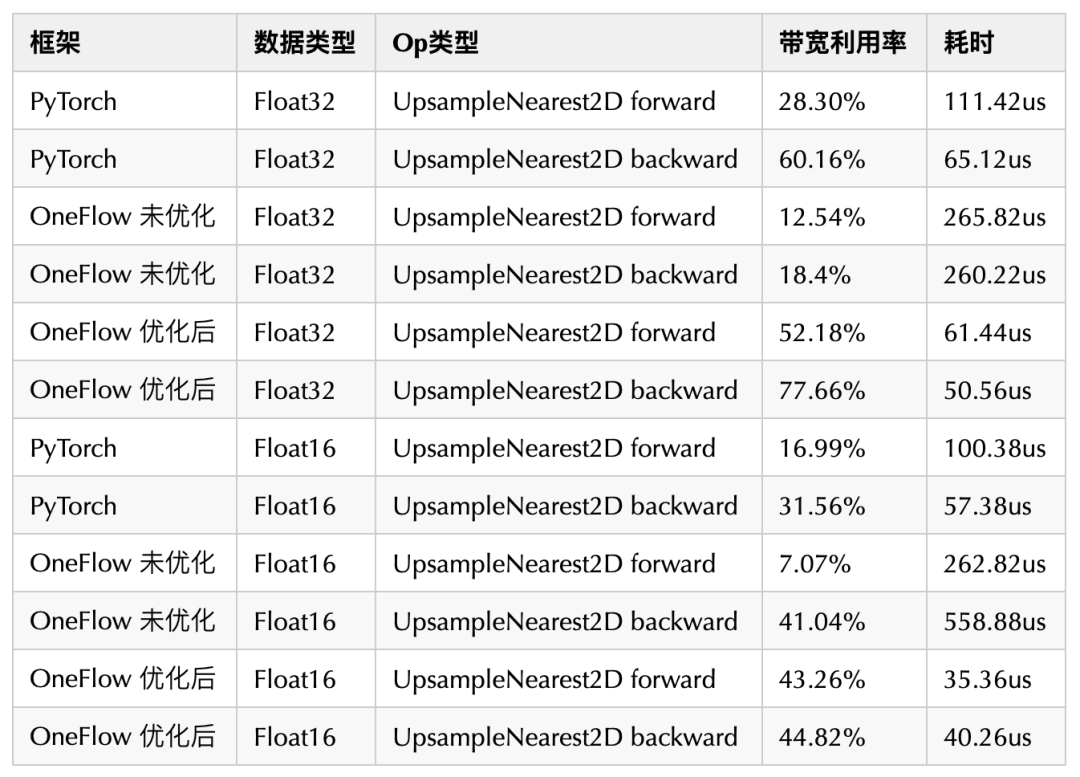• 为常见的情况写特例，比如这里就是为采样倍数为2的Nearest插值写特例，避免使用NdIndexHelper带来的额外计算开销，不用追求再一个kernel实现中同时拥有通用型和高效性；

• 整数除法开销大（但是编译器有的时候会优化掉一些除法），nchw中的nc不需要分开，合并在一起计算减少计算量；

• int64_t除法的开销更大，用int32满足大部分需求，其实这里还有一个快速整数除法的问题；

• 反向Kernel计算过程中循环dx相比循环dy ，实际上将坐标换算的开销减少到原来的1/4；

• CUDA GMEM的开销的也比较大，虽然编译器有可能做优化，但是显式的使用局部变量更好；

• 一次Memset的开销也很大，和写一次一样，所以反向Kernel中对dx使用Memset清零的时机需要注意；

• 向量化访存。

### 2.2 对bbox_iou函数进行优化 (垂直Fuse优化)

````def bbox_iou(box1, box2, xywh=True, GIoU=False, DIoU=False, CIoU=False, eps=1e-7):``    # Returns Intersection over Union (IoU) of box1(1,4) to box2(n,4)`
`    # Get the coordinates of bounding boxes``    if xywh:  # transform from xywh to xyxy``        (x1, y1, w1, h1), (x2, y2, w2, h2) = box1.chunk(4, -1), box2.chunk(4, -1)``        w1_, h1_, w2_, h2_ = w1 / 2, h1 / 2, w2 / 2, h2 / 2``        b1_x1, b1_x2, b1_y1, b1_y2 = x1 - w1_, x1 + w1_, y1 - h1_, y1 + h1_``        b2_x1, b2_x2, b2_y1, b2_y2 = x2 - w2_, x2 + w2_, y2 - h2_, y2 + h2_``    else:  # x1, y1, x2, y2 = box1``        b1_x1, b1_y1, b1_x2, b1_y2 = box1.chunk(4, -1)``        b2_x1, b2_y1, b2_x2, b2_y2 = box2.chunk(4, -1)``        w1, h1 = b1_x2 - b1_x1, (b1_y2 - b1_y1).clamp(eps)``        w2, h2 = b2_x2 - b2_x1, (b2_y2 - b2_y1).clamp(eps)`
`    # Intersection area``    inter = (b1_x2.minimum(b2_x2) - b1_x1.maximum(b2_x1)).clamp(0) * \``            (b1_y2.minimum(b2_y2) - b1_y1.maximum(b2_y1)).clamp(0)`
`    # Union Area``    union = w1 * h1 + w2 * h2 - inter + eps`
`    # IoU``    iou = inter / union``    if CIoU or DIoU or GIoU:``        cw = b1_x2.maximum(b2_x2) - b1_x1.minimum(b2_x1)  # convex (smallest enclosing box) width``        ch = b1_y2.maximum(b2_y2) - b1_y1.minimum(b2_y1)  # convex height``        if CIoU or DIoU:  # Distance or Complete IoU https://arxiv.org/abs/1911.08287v1``            c2 = cw ** 2 + ch ** 2 + eps  # convex diagonal squared``            rho2 = ((b2_x1 + b2_x2 - b1_x1 - b1_x2) ** 2 + (b2_y1 + b2_y2 - b1_y1 - b1_y2) ** 2) / 4  # center dist ** 2``            if CIoU:  # https://github.com/Zzh-tju/DIoU-SSD-pytorch/blob/master/utils/box/box_utils.py#L47``                v = (4 / math.pi ** 2) * (torch.atan(w2 / h2) - torch.atan(w1 / h1)).pow(2)``                with torch.no_grad():``                    alpha = v / (v - iou + (1 + eps))``                return iou - (rho2 / c2 + v * alpha)  # CIoU``            return iou - rho2 / c2  # DIoU``        c_area = cw * ch + eps  # convex area``        return iou - (c_area - union) / c_area  # GIoU https://arxiv.org/pdf/1902.09630.pdf``    return iou  # IoU````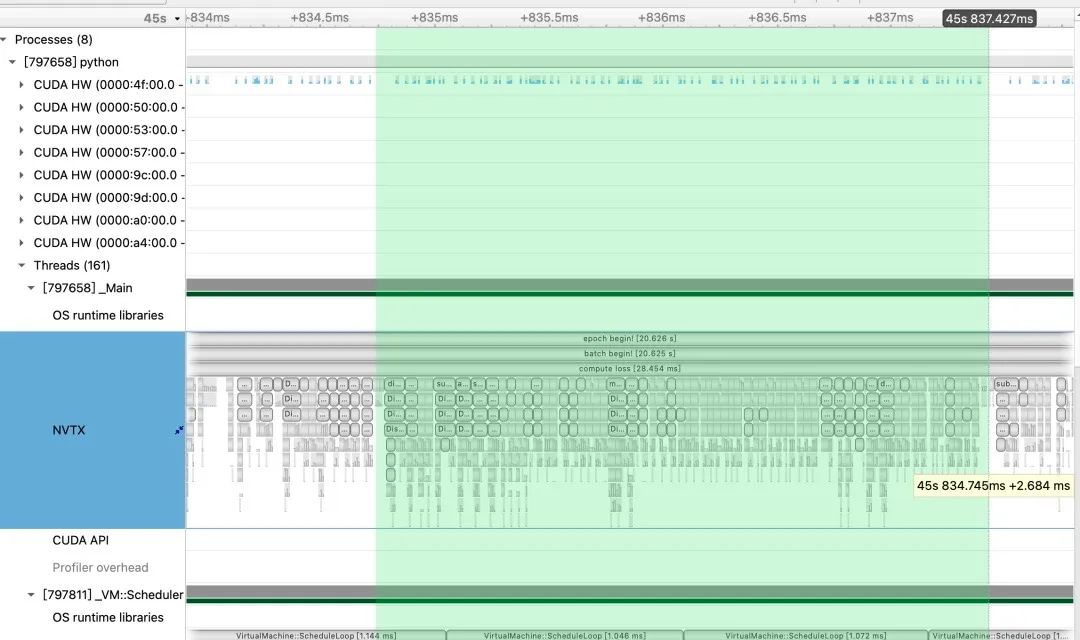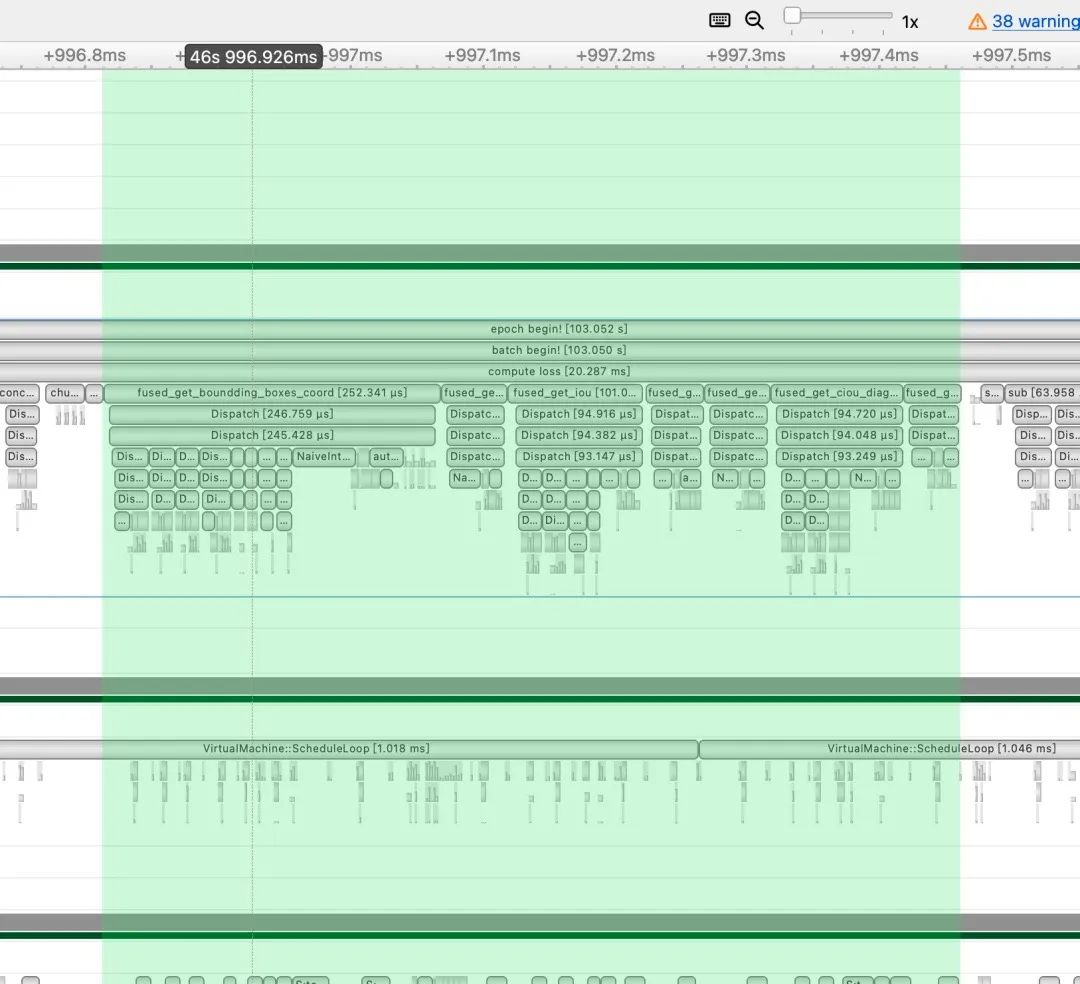• fused_get_boundding_boxes_coord：https://github.com/Oneflow-Inc/oneflow/pull/9433

• fused_get_intersection_area: https://github.com/Oneflow-Inc/oneflow/pull/9485

• fused_get_iou: https://github.com/Oneflow-Inc/oneflow/pull/9475

• fused_get_convex_diagonal_squared: https://github.com/Oneflow-Inc/oneflow/pull/9481

• fused_get_center_dist: https://github.com/Oneflow-Inc/oneflow/pull/9446

• fused_get_ciou_diagonal_angle: https://github.com/Oneflow-Inc/oneflow/pull/9465

• fused_get_ciou_result: https://github.com/Oneflow-Inc/oneflow/pull/9462

### 2.3 对模型滑动平均更新进行优化（水平Fuse优化）

````def update(self, model):``        # Update EMA parameters``        self.updates += 1``        d = self.decay(self.updates)`
`        msd = de_parallel(model).state_dict()  # model state_dict``        for k, v in self.ema.state_dict().items():``            if v.dtype.is_floating_point:  # true for FP16 and FP32``                v *= d``                v += (1 - d) * msd[k].detach()``        # assert v.dtype == msd[k].dtype == flow.float32, f'{k}: EMA {v.dtype} and model {msd[k].dtype} must be FP32'````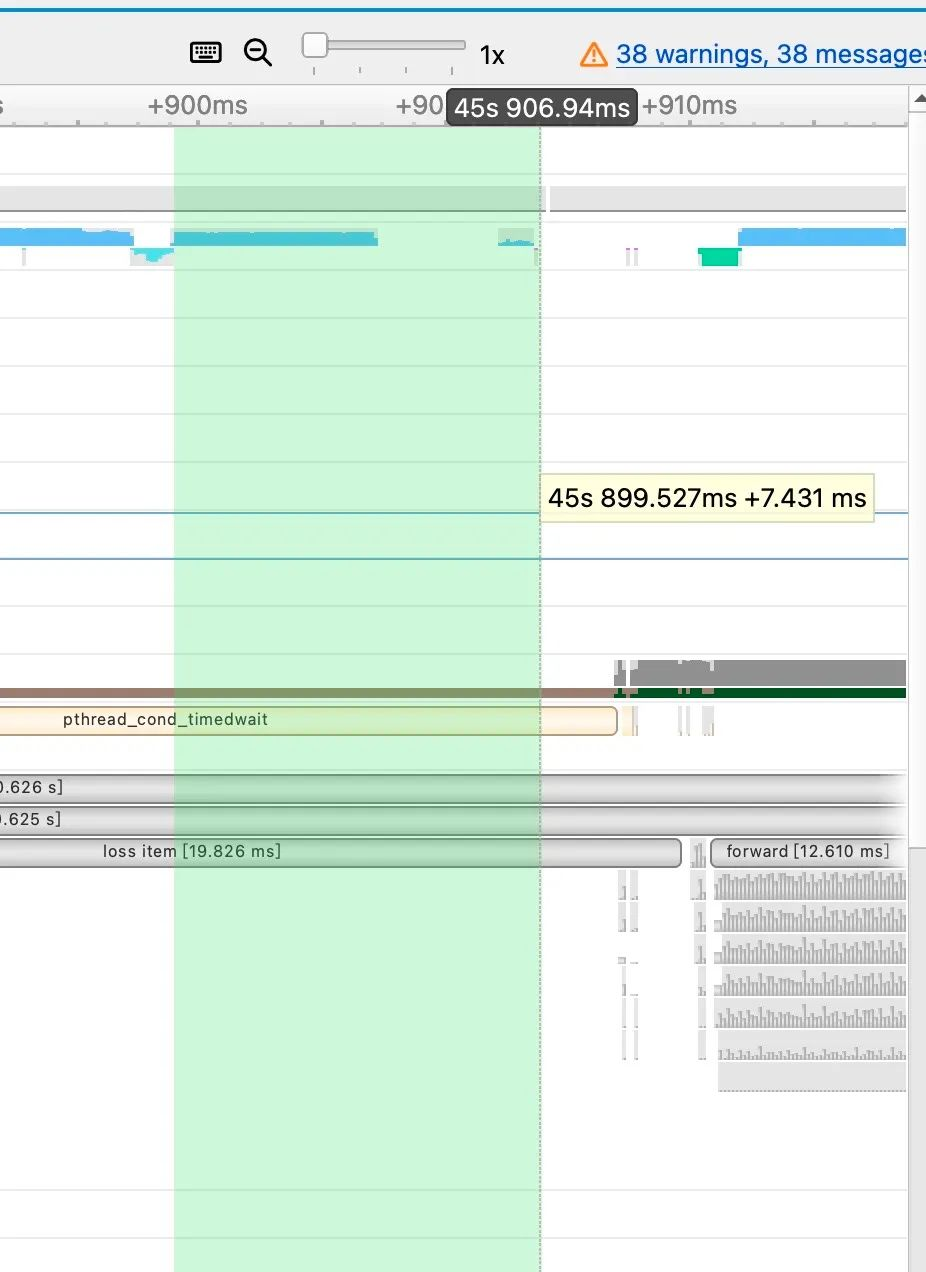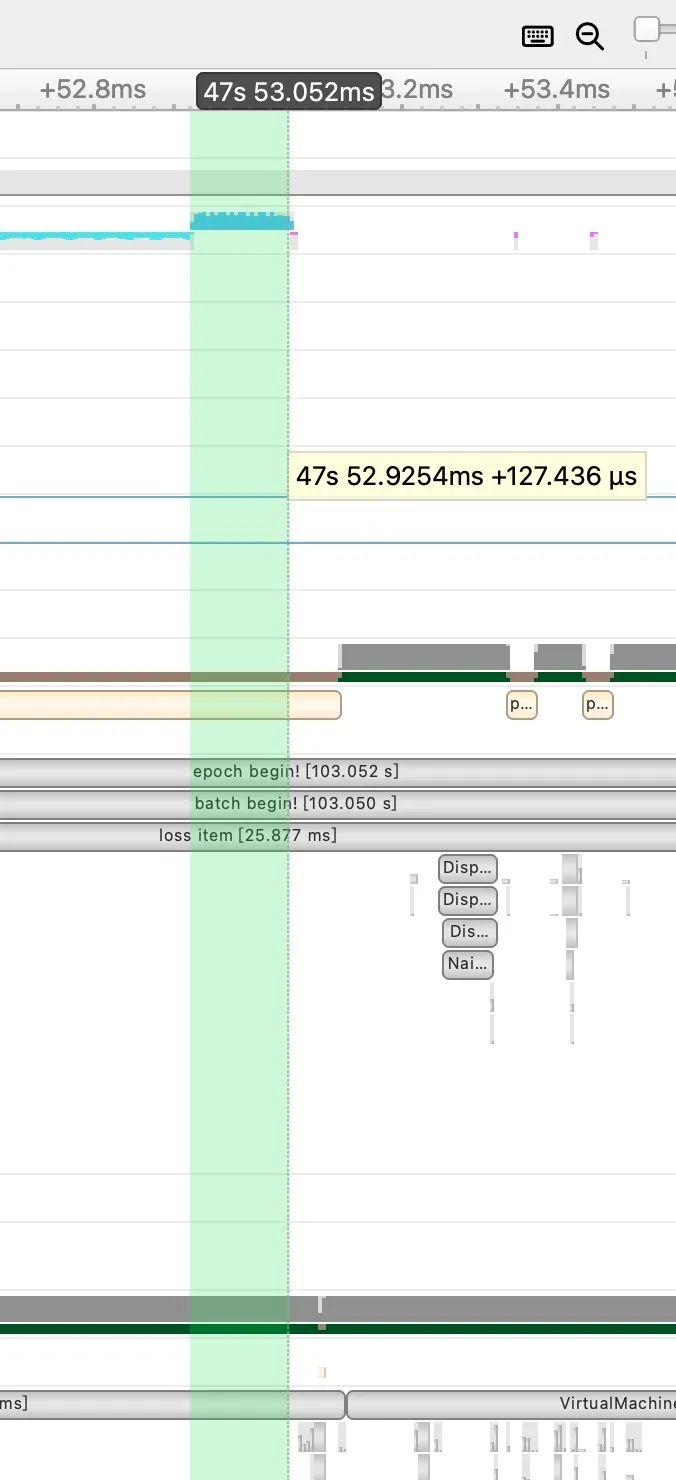## 使用方法

``python train.py ``--batch 16 ``--cfg models/yolov5s.yaml ``--weights '' ``--data coco.yaml ``--img 640 ``--device 0 ``--epoch 1 ``--bbox_iou_optim ``--multi_tensor_optimizer``

## 总结

https://github.com/Oneflow-Inc/one-yolov5

One-YOLOv5的优化工作实际上不仅包含性能，我们目前也付出了很多心血在文档和源码解读上，后续会继续放出《YOLOv5全面解析教程》的其他文章，并将尽快发布新版本。

## 欢迎Star、试用OneFlow最新版本：https://github.com/Oneflow-Inc/oneflow/